#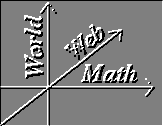World Web Math: Vector Calculus Summary

Single variable calculus studied how to take limits, derivatives, and integrals of single variable functions like f(x) = sin x. Multivariable or vector calculus studies how to take limits, derivatives, and integrals of multivariable functions of the sort## Vectors

Vector calculus starts out, appropriately enough, with vectors: those quantities, often denoted by arrows, that have a magnitude and direction but perhaps no fixed location.

(For much of these pages, vectors will be denoted by either a bold symbol, such as v for a generic vector or i for a unit vector when these quantities appear in the text. Overarrows may be used in displayed equations as well as the boldface symbols.)

If we adopt a cartesian coordinate system, then a three dimensional vectormay be reprsented as the 3-tuple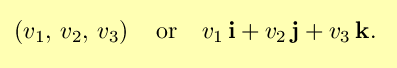You can add vectors and multiply them by scalars: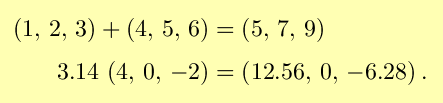The magnitude of a vector v is denoted byand is equal to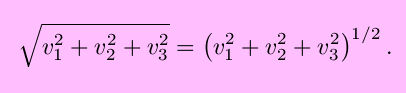by the Pythagorean theorem, with similar forms for a higher or lower number of dimensions. A unit vector is a vector with magnitude 1, and any nonzero vector can be made into a unit vector by dividing by its magnitude.

There are two ways to multiply vectors: the dot product and the cross product. The two ways are quite different, and a major part of learning vector calculus is appreciating the difference. The dot product of two vectors v and w is the scalar value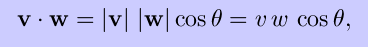where the angle ``theta'' is the angle between v and w. Two nonzero vectors are orthogonal (perpendicular) if and only if their dot product is zero. It can be shown that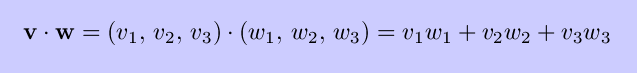and that the projection of v onto w isThe cross product of two three-dimensional vectors is another vector, denotedThis vector has magnitude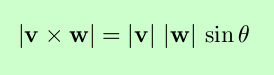and direction orthogonal to the two vectors v and w, with orientation given by the right-hand rule. Two nonzero vectors are parallel if and only if their cross product is zero. Note that the magnitude of the cross product is also the area of the parallelogram with sides v and w. It can be shown that the cross product in 3-tuple form is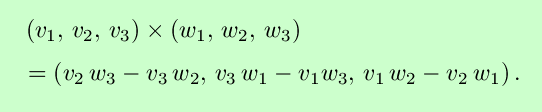Vectors make it easier to describe our favorite geometric objects. For example, lines in three-space can be written parametrically aswhere P is a point on the line and v is a nonzero vector parallel to the line.

The plane with normal vector n that contains the point Q can be described implicitly by the equation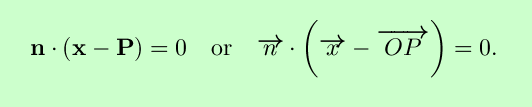Cartesian coordinates are not the only way to label points in three-dimensional space. You might recall polar coordinates, which associated the pair of coordinatesto each point in the plane, where the first coordinate is the distance from the origin and the second is the angle with respect to the positive x-axis. There are two different ways of generalizing polar coordinates to three dimensions: cylindrical and spherical coordinates. In cylindrical coordinates, each point has an r and a theta coordinate obtained by projecting the point onto the x-y plane and using the polar coordinates of the projection, plus a z coordinate which is the same as in cartesian coordinates. In spherical coordinates, every point is assigned three coordinates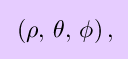where the first (``rho'') is the distance from the origin, the second (``theta'', as in plane polar coordinates) is the angle that the vector from the origin to the point makes with the x-z plane, and the third (``phi'') is the angle that the vector makes with the positive z-axis.

## Vector Calculus at A Glance

Now that we have vectors, we can use them as the input and output of certain operators.. The following table summarizes the sorts of objects we get when we do this, and what their derivatives and integrals are called. The column labeled ``Function'' gives the domain and range of the type of function considered.

Function Notation Name Derivatives Integrals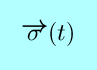curvepath integral, line integralscalar fielddouble, triple, path and surface integralsparameterized surface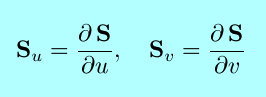surface integral, flux integral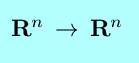vector field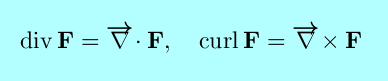line integral, flux integral

## Scalar-valued functions

• Sections and level sets
• Graphing scalar valued functions
• Limits
• Continuity
• Directional derivatives
• Partial derivatives
• Total differentials
• The Derivative Matrix
• Tangent planes
• Linear approximation
• Differentiability
• The Simple Chain Rule
• The Matrix Chain Rule
• The Paths Chain Rule
• Iterated partial derivatives

## Maximization and Minimization

To find the maximum and minimum points of a scalar valued function f(x) on a domain D, follow this procedure:
1. Look at all the critical points x where the gradient of f(x) = 0. Throw out any that aren't in D.
2. Look at any points where f isn't differentiable.
3. Look at the boundary of D. You can this either by
• Parameterizing the boundary so that you have an unconstrained max/min problem, or by
• Lagrange multipliers: if the boundary of D is described as a level set by g(x) = 0, then the critical points of f constrained to be on the boundary of D can be found by solving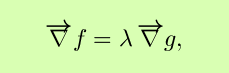4. If x is the critical point in an unconstrained max/min problem, you can determine whether it is a local max, local min, or saddle point by looking at the Hessian.

## Vector Fields

A vector field is a function which associates a vector to every point in space. You can graph a vector field (for n=2) by picking lots of points (preferably some in each quadrant), evaluating the vector field at these points, and then drawing the resulting vector with its tail at the point. You may also want to indicate flow lines, which are paths whose velocity vector at a point is the same as the value of the vector field at that point.

There are two derivatives which are useful in examining the local geometry of a vector field with n=2 or 3.

The divergence of F(x,y,z) is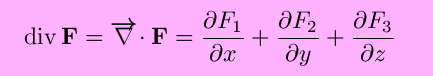(only the first two terms if n=2) and measures the extent to which the point is a ``source'' or ``sink'' of the vector field.

The curl of F(x,y,z) is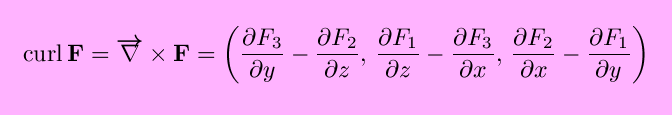(only the z-component is nonzero if n=2) and measures the ``rotation'', or ``circulation per unit area'', of the vector field at a point.

Of special interest are gradient vector fields, those vector fields that are the gradient of some scalar potential function. Gradient vector fields are also called conservative vector fields, because the work done by a particle moving in a closed loop against a gradient vector field is always 0. The curl of a gradient vector field is the zero vector; this is useful in testing whether an arbitrary vector field is conservative. Symbolically,## Double and Triple Integrals

• Cavalieri's Principle
• Definition of Double Integral
• Fubini's Theorem
• Iterated Integrals
• Double Integrals over General Regions
• Changing the Order of Integration
• Triple Integrals
• Applications of Double and Triple Integrals
• Change of Variables

## Surfaces

The graph of a function of two variables is a surface, and the set of points in three-space satisfying a single equation in general is a surface, but the most useful and most general definition of a surface is as a mapping from a two dimensional region into three space. [Think of the straight longitude and latitude lines on a flat map being sent to the curved longitude and latitude lines on a sphere.]
• Surface Area
• Scalar Surface Integrals
• Surface Flux Integrals

## Fundamental Theorems of Vector Calculus

(While this page is under construction, you might want to take a look at the theorems presented together, at Fundamental Theorems of Calculus, complete with figures in gaudy colors.)
• FTC for Work Integrals
• Green's Theorem
• Stokes' Theorem
• Gauss' Theorem

Vector Calculus Index | World Web Math Categories Page

watko@athena.mit.edu